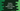# Working with random in python , generate a number,float in range etc.## How to generate a random number in python :

In this tutorial, we will learn how to create a random number _in python. Generating a random number in python is easier than you think. Everything is already defined in a module called _random. Just import it and use its inbuilt functions.

I will show you how to print a_ random float, _random float in a range, random integer, random integer in a range, even random number in a range, random element in a sequence and how to shuffle a list.

All the code in this tutorial is compatible with python 3. If you have python 2 installed on your system, maybe you will have to change a few lines.

``````#  Print a random float

import random

print(random.random())

#  Print a random float in range

import random

print(random.uniform(1,10))

# Print a random integer
import random

print(random.randrange(10))

# Print an integer number within a range
import random

print("Random number using randrange : ");
print(random.randrange(2,10))

print("Random number using randint : ");
print(random.randint(2,10))

# Print only even random number in a range
import random

print("Even Random number : ")
print(random.randrange(2,10,2))

print("Random number Divisible by 5 : ")
print(random.randrange(0,100,5))

# Print a random element in a sequence
import random

days = ["sun","mon","tue","wed","thu","fri","sat"]
print(random.choice(days))

# Shuffle a list
import random

days = ["sun","mon","tue","wed","thu","fri","sat"]

for x in range(5):
print("shuffling..")
random.shuffle(days)
print(days)``````

## Example 1: Print a random float :

Don’t forget to import the ’random’ module at the start of the program.

The method_ ‘random()’_ is called to create one random float.

## Example 2: Print a random float in a range :

It will print one random number within 1 and 10. uniform() method takes two arguments and returns one random number within the range. This method is useful if you want to get a random number in a range.

## Example 3: Print a random integer :

It will print an integer from_ 0 to 9_. Run it and you will always get one value in this range. You can use this method to set the upper range of the generated random number.

## Example 4: Print an integer number within a range :

To print an integer in a given range, we have two functions : randrange(a,b) and randint(a,b). The only difference between both is that randint(a,b) will include all integers from a to b, but randrange(a,b) will include integers from a to b-1. That means_ randint(a,b)_ is similar to randrange(a,b+1). Let’s have a look at how to implement both :

Keep running it continuously. At one point, randint will print 10, but randrange will never.

## Example 5: Print only even random number in a range :

We can pass one more argument to the randrange function. It is known as step i.e. if we pass 2, it will produce only numbers divisible by 2, for 3 it will produce numbers divisible by 3 etc. Let’s take a look :

The first one will print only even random numbers in between 2 to 9 and the second one will print only random numbers divisible by 5 in between 0 to 99. ’randrange’ is a useful method if we want to produce random integers. Using this method, we can create one random integer less than a number, random integer within a range or a random integer divisible by a specific number.

## Example 6: Print a random element in a sequence :

The random module contains one method called choice that takes one sequence as the argument and returns one random element of that sequence. If the sequence is empty, it will raise one IndexError.Let’s take a look at the below program to understand this :

It will print one random word from the provided input sequence.

## Example 7: Shuffle a list :

Module random also has one method called shuffle that shuffles all elements of a list. For example :

It will print output like below :

__ View on Github